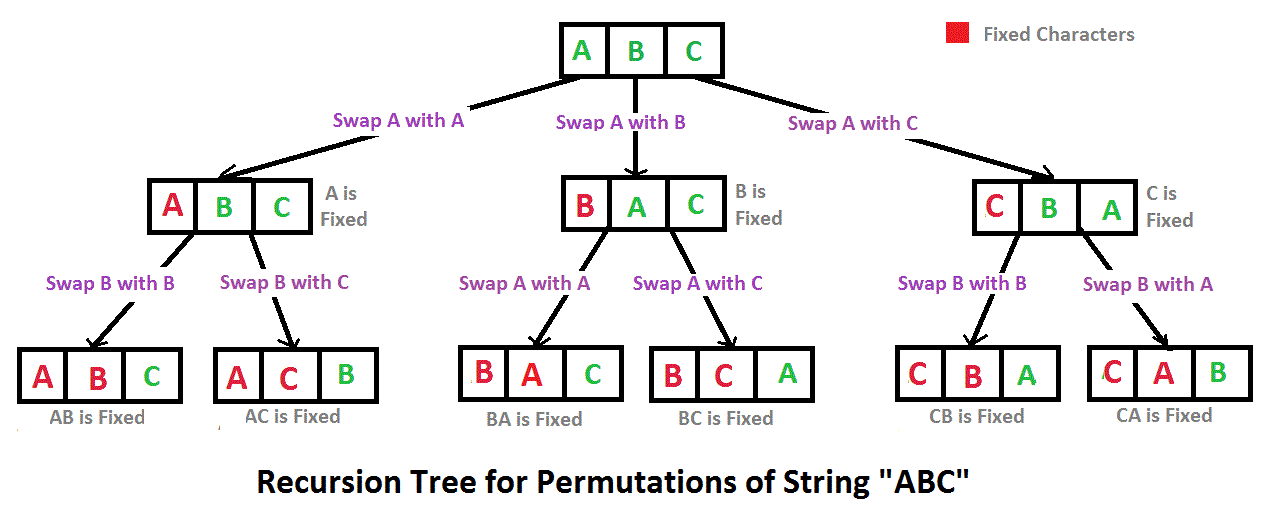Open In App

# C++ Program To Print All Permutations Of A Given String

A permutation also called an “arrangement number” or “order,” is a rearrangement of the elements of an ordered list S into a one-to-one correspondence with S itself. A string of length n has n! permutation.

Source: Mathword(http://mathworld.wolfram.com/Permutation.html)

Below are the permutations of string ABC.
ABC ACB BAC BCA CBA CAB

Here is a solution that is used as a basis in backtracking.## C++

 `// C++ program to print all permutations ``// with duplicates allowed ``#include  ``using` `namespace` `std; `` ` `// Function to print permutations ``// of string ``// This function takes three parameters: ``// 1. String ``// 2. Starting index of the string ``// 3. Ending index of the string. ``void` `permute(string a, ``int` `l, ``int` `r) ``{ ``    ``// Base case ``    ``if` `(l == r) ``        ``cout<

Output:

```ABC
ACB
BAC
BCA
CBA
CAB```

Time Complexity: O(n*n!) Note that there are n! permutations and it requires O(n) time to print a permutation.

Auxiliary Space: O(r – l)

Note: The above solution prints duplicate permutations if there are repeating characters in the input string. Please see the below link for a solution that prints only distinct permutations even if there are duplicates in input.
Print all distinct permutations of a given string with duplicates.
Permutations of a given string using STL

Another approach:

## C++

 `// C++ program to implement``// the above approach``#include ``#include ``using` `namespace` `std;`` ` `void` `permute(string s, ``             ``string answer)``{``    ``if``(s.length() == 0)``    ``{``        ``cout << answer << ``"  "``;``        ``return``;``    ``}``    ``for``(``int` `i = 0; ``            ``i < s.length(); i++)``    ``{``        ``char` `ch = s[i];``        ``string left_substr = s.substr(0, i);``        ``string right_substr = s.substr(i + 1);``        ``string rest = left_substr + right_substr;``        ``permute(rest , answer+ch);``    ``}``}`` ` `// Driver code``int` `main()``{``    ``string s;``    ``string answer = ``""``;`` ` `    ``cout << ``"Enter the string : "``;``    ``cin >> s;`` ` `    ``cout << ``    ``"All possible strings are : "``;``    ``permute(s, answer);``    ``return` `0;``}`

Output:

```Enter the string : abc
All possible strings are : abc  acb  bac  bca  cab  cba```

Time Complexity: O(n*n!) The time complexity is the same as the above approach, i.e. there are n! permutations and it requires O(n) time to print a permutation.

Auxiliary Space: O(|s|)

Please refer complete article on Write a program to print all permutations of a given string for more details!